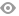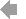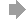Basic HTML VersionView Full Version
Page 38 - Course Selection Guide 2021-2022
P. 38

ALGEBRA I (552)
This course is the standard course for the majority of students. Emphasis is placed on acquiring a solid knowledge of the fundamentals of algebra. Topics include: uses of algebra, operations with real numbers, solving linear equations and inequalities, graphing relations and functions, systems of equations and inequalities, absolute values, exponents and polynomials, rational expressions, word problems with rational expressions, radicals, quadratic functions and equations, and descriptive statistics.
HONORS ALGEBRA I/GEOMETRY (512)
Year 1 Credit Grade 9 Req: Department approval
This course is the first year of a two-year fast-tracked mathematics sequence covering the Honors level Algebra I, Geometry, and Algebra II courses. It is intended for the superior mathematics student who is interested in finishing more than four math courses in high school. The student will work at an accelerated pace and in more depth to finish all of Algebra I and more than half of Geometry in one year.
HONORS GEOMETRY (556)
Year 1 Credit Grade 9 Req: Department approval
This course is intended for the student with excellent mathematics ability who has successfully completed a bona fide Algebra I course in 8th grade. The emphasis is on geometric relationships using algebraic techniques. Topics covered include an Algebra review, ACT and SAT strategies, and the topics in regular geometry, plus proofs, logic, fractals, and other geometric topics. The students will also work with computer technology to explore geometric concepts in the classroom.
GEOMETRY (521)
Year 1 Credit Grades 9, 10, 11, 12 Req: Algebra I; Algebra IB with Department approval. Grade 9 requires Department approval.
This course is the standard grade 10 course for the majority of students. Emphasis is on geometric relationships using algebraic techniques. Topics covered include introduction to geometry, introduction to proof, congruent triangles, parallel lines, using parallel lines, similarity, right triangles, circles, area, surface area, volume, coordinate geometry, and conditional probability. Incoming freshmen who have successfully completed a bona fide Algebra I course in eighth grade and who have met the Algebra I criteria at Padua Franciscan High School may take this course.
GEOMETRY: MATH 11 (521 S)
Year 1 Credit Grade 11 Req: Qualifying learning disability and appropriate IEP
This course is fully equivalent to the Geometry course (521) in focus and in content. It is designed to meet the needs of students who have been identified with a specified learning disability in mathematical computation and/or mathematical reasoning. Topics covered include introduction to Geometry, introduction to proof, congruent triangles, parallel and perpendicular lines, similarity, right triangles, circles, area, surface area, volume, coordinate geometry, and conditional probability. Course assignments, activities, and assessments will be differentiated according to student needs.
3736   37   38   39   40